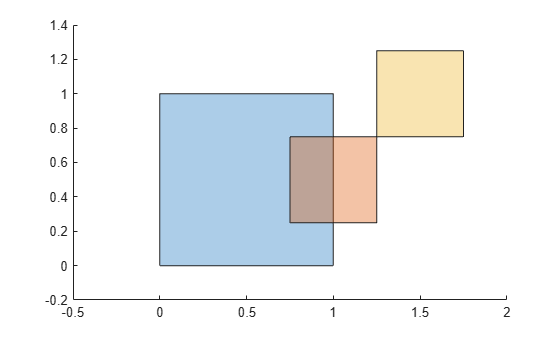# overlaps

Determine whether `polyshape` objects overlap

## Syntax

``TF = overlaps(poly1,poly2)``
``TF = overlaps(polyvec)``

## Description

````TF = overlaps(poly1,poly2)` returns a logical array whose elements are 1 when the corresponding element pairs of two `polyshape` arrays with compatible sizes overlap. TF(i,j) is 1 when the ith `polyshape` in `poly1` overlaps the jth `polyshape` in `poly2`.```

example

````TF = overlaps(polyvec)` returns a logical array whose elements are 1 (`true`) when the corresponding element pairs of a vector of `polyshape` objects overlap. TF(i,j) is 1 when the ith `polyshape` in `polyvec` overlaps the jth `polyshape`.```

## Examples

collapse all

Create and plot a 1-by-3 vector of polygons, then determine which pairs of polygons overlap.

```p1 = polyshape([0 0 1 1],[1 0 0 1]); p2 = polyshape([0.75 1.25 1.25 0.75],[0.25 0.25 0.75 0.75]); p3 = polyshape([1.25 1.25 1.75 1.75],[0.75 1.25 1.25 0.75]); polyvec = [p1 p2 p3]; plot(polyvec)````TF = overlaps(polyvec)`
```TF = 3x3 logical array 1 1 0 1 1 0 0 0 1 ```

Since the third polygon does not overlap either the first or second polygon, `TF(1,3)`, `TF(2,3)`, `TF(3,1)`, and `TF(3,2)` are 0. The single point shared by the second and third polygon is not considered an overlapping region.

## Input Arguments

collapse all

First input `polyshape`, specified as a scalar, vector, matrix, or multidimensional array.

Second input `polyshape`, specified as a scalar, vector, matrix, or multidimensional array.

`polyshape` vector.

## Output Arguments

collapse all

Overlap indicator, returned as a scalar, vector, matrix, or multidimensional array.

• If you input two `polyshape` arguments, then they must have compatible sizes. For example, if two `polyshape` vectors have different lengths M and N, then they must have different orientations (one must be a row vector and one must be a column vector). `TF` is then M-by-N or N-by-M depending on the orientation of each input vector. For more information on compatible array sizes, see Compatible Array Sizes for Basic Operations.

• If you input a single `polyshape` vector with length N, then `TF` is N-by-N.

Data Types: `logical`

## Version History

Introduced in R2018a